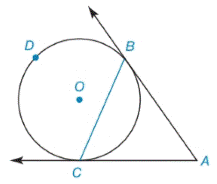Chapter 6.2, Problem 9E### Elementary Geometry for College St...

6th Edition
Daniel C. Alexander + 1 other
ISBN: 9781285195698

#### Solutions

Chapter
Section### Elementary Geometry for College St...

6th Edition
Daniel C. Alexander + 1 other
ISBN: 9781285195698
Textbook Problem
19 views

# Given: Tangent A B →  and A C → to ⊙ O m ∠ A C B = 68 ° Find: a) m B C ⌢ b) m B D C ⌢ c) m ∠ A B C d) m ∠ ATo determine

(a)

To find:

mBC when AB and AC are tangent to O, mACB=68°.

Explanation

Corollary:

The measure of an angle formed by a tangent and a chord drawn to the point of tangency is one-half the measure of the two intercepted arc.

Calculation:

mBC when AB and AC are tangent to O, mACB=68°.

To determine

(b)

To find:

mBDC when AB and AC are tangent to O, mACB=68°.

To determine

(c)

To find:

mABC when AB and AC are tangent to O, mBC=136°.

To determine

(d)

To find:

mA when AB and AC are tangent to O, mBC=136° and mBDC=224°.

### Still sussing out bartleby?

Check out a sample textbook solution.

See a sample solution

#### The Solution to Your Study Problems

Bartleby provides explanations to thousands of textbook problems written by our experts, many with advanced degrees!

Get Started

#### Solve the equations in problem 1-6. 2.

Mathematical Applications for the Management, Life, and Social Sciences

#### Evaluate the integral. 19. z3ezdz

Single Variable Calculus: Early Transcendentals

#### Multiply. (2cos+3)(4cos5)

Trigonometry (MindTap Course List)

#### True or False: If converges absolutely, then it converges conditionally.

Study Guide for Stewart's Single Variable Calculus: Early Transcendentals, 8th

#### In Exercises 116, determine whether the argument is valid. pqqrpr

Finite Mathematics for the Managerial, Life, and Social Sciences